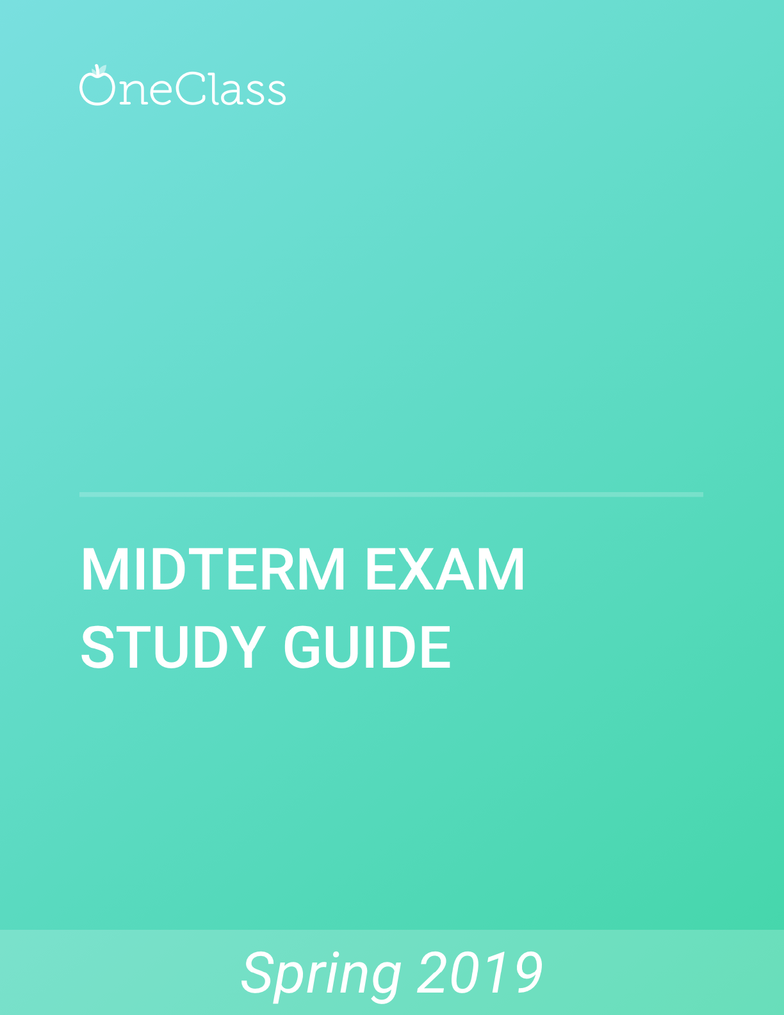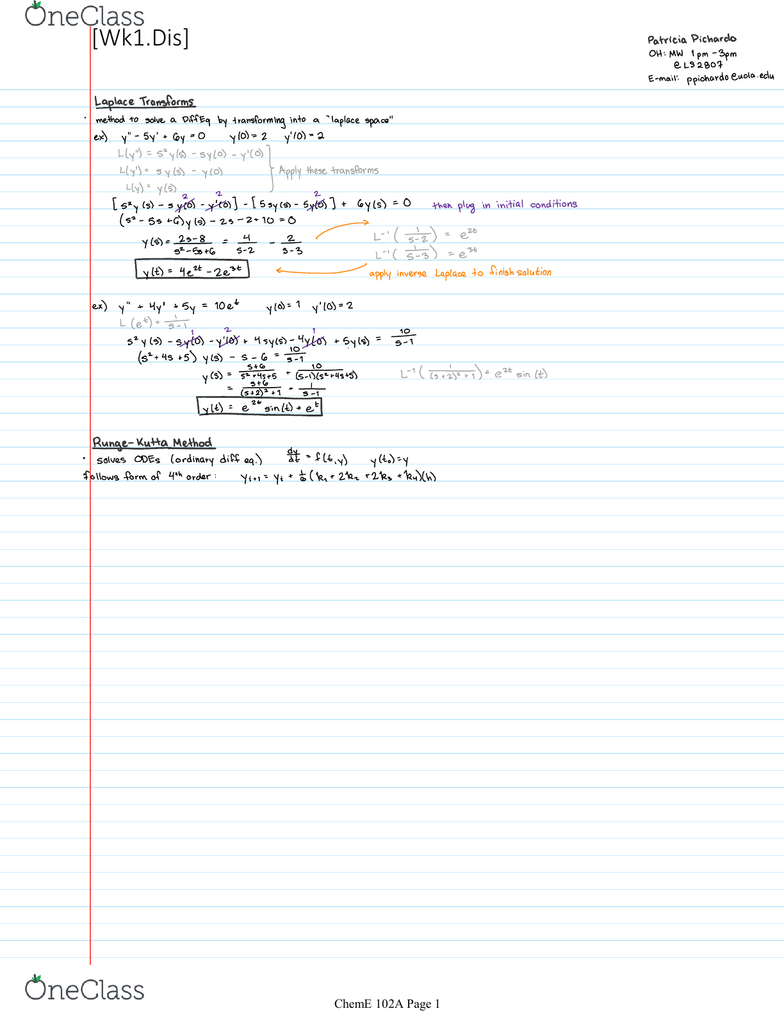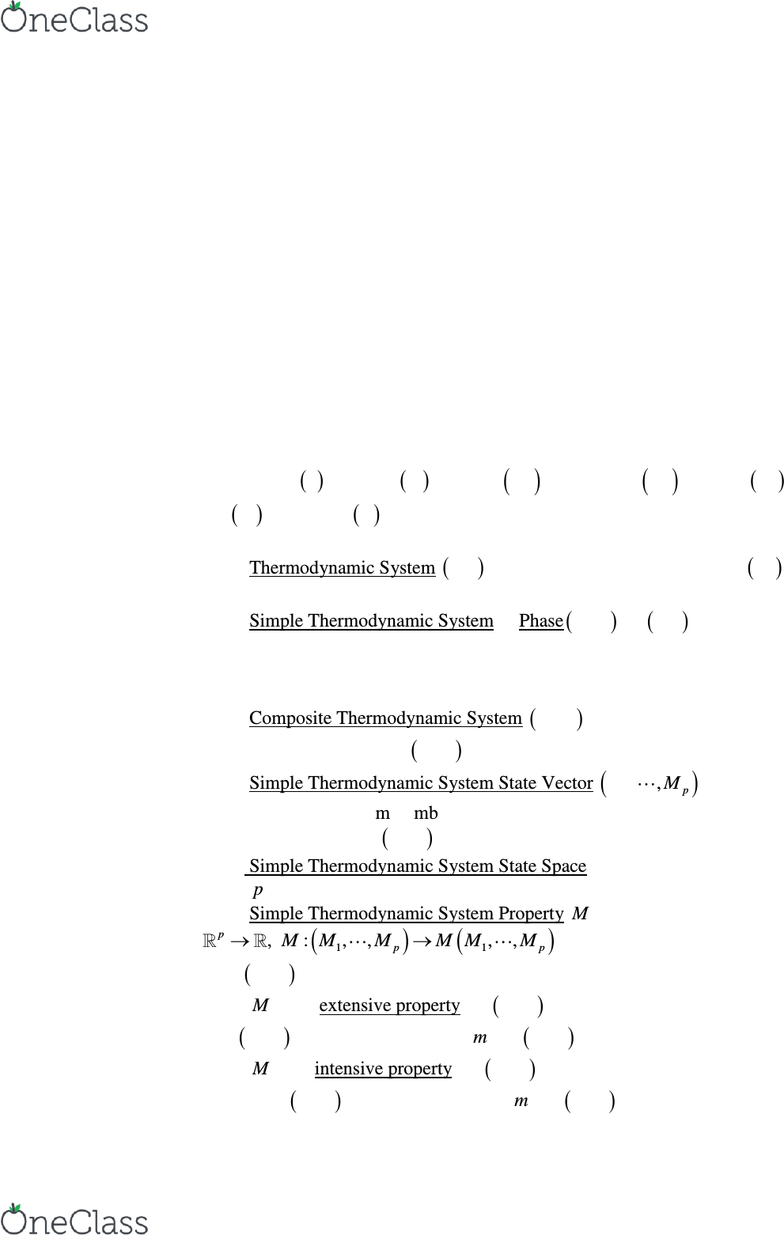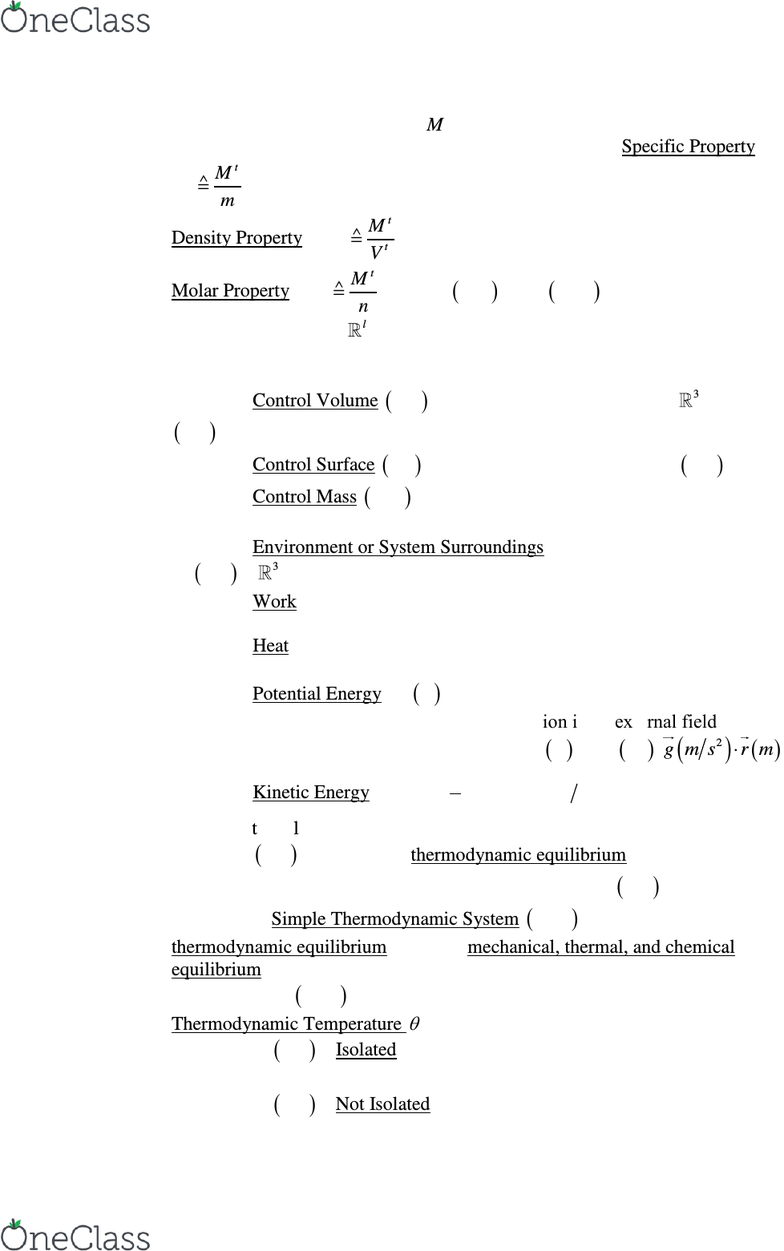# CH ENGR 102A Study Guide - Winter 2019, Comprehensive Midterm Notes - Thermodynamics, Ideal Gas, Entropy

120 views327 pagesCH ENGR 102A
Unlock document

This preview shows pages 1-3 of the document.
Unlock all 327 pages and 3 million more documents.[Wk1.Dis]
Friday, January 13, 2017 9:00 AM
ChemE 102A Page 1
find more resources at oneclass.com
find more resources at oneclass.com
Unlock document

This preview shows pages 1-3 of the document.
Unlock all 327 pages and 3 million more documents.ChE 102A, Thermodynamics I, Winter 2017
Instructor Vasilios I. Manousiouthakis
“ἓν οδα, τι οδν οδα
Σωκράτης
Axiomatic Approach to Thermodynamics
The axiomatic approach to Thermodynamics is built through the introduction of Axioms
which are considered to be true statements, Definitions, and Theorems which are derived
through the use of axioms, definitions, and previously developed theorems. These
concepts are presented in the order they are introduced or developed, and are numbered
in the same order. Axioms, Definitions, and Theorems are indicated with an A, D, T,
respectively following their number.
1. A. Time
ts
, Length
lm
, Area
2tm
, Volume
3t
Vm
, Mass
,
Force
FN
, Energy
t
EJ
are undefined primitive concepts whose meaning is
considered known
2. D. Thermodynamic System
Sys
is a given finite amount of mass
under consideration
3. D. Simple Thermodynamic System or Phase
SSys
is a
Sys
that is
macroscopically homogeneous, isotropic, uncharged, not acted upon by electric,
magnetic, and gravitational fields, and sufficiently large so that surface effects are
negligible
4. D. Composite Thermodynamic System
CSys
comprises of at least two
simple thermodynamic systems
SSys
5. D. Simple Thermodynamic System State Vector
1,,p
MM
is a vector
which contains the minimum number of variables, knowledge of the values of
which uniquely determines
SSys
behavior of interest
6. D. Simple Thermodynamic System State Space is the linear space of
dimension
p
, whose points are simple thermodynamic system state vectors
7. D. Simple Thermodynamic System Property
M
is a function
11
: , : , , , ,
p
pp
M M M M M M M
whose values describe some
aspect of
SSys
behavior of interest
8. D.
t
M
is an extensive property of
SSys
iff its values vary with the extent
(size) of
SSys
and are proportional to
m
of
SSys
9. D.
M
is an intensive property of
SSys
iff its values do not vary with the
extent (size) of
SSys
and do not depend on
m
of
SSys
find more resources at oneclass.com
find more resources at oneclass.com
Unlock document

This preview shows pages 1-3 of the document.
Unlock all 327 pages and 3 million more documents.10. D. An extensive property
t
M
can give rise to several intensive properties
through appropriate scaling. They are defined as follows: Specific Property is
t
M
Mm
Density Property is
t
t
M
MV
Molar Property is
t
M
Mn
, where
n mol
is the
SSys
number of moles
11. D. A subset of
l
is an open connected set iff there do not exist two
nonempty open subsets of this set whose union is the set and whose intersection is
the empty set
12. D. Control Volume
CV
is the open connected subset of
3
occupied by
Sys
at some time
0
t
13. D. Control Surface
CS
is the boundary of the closure of
CV
14. D. Control Mass
CM
is a given finite amount of mass surrounded by
real or imaginary boundaries that cannot be crossed by the considered mass
15. D. Environment or System Surroundings is the complement of the closure
of
CV
in
3
16. D. Work is energy in transit to a system’s macroscopically observable
motion coordinates
17. D. Heat is energy in transit to a system’s macroscopically unobservable
motion coordinates
18. D. Potential Energy
P
EJ
is the part of a system’s energy that can lead to
work generation solely due to the system’s position in an external field. If the
external field is the earth’s gravity field, then
2
P
E J m kg g m s r m 
19. D. Kinetic Energy
 
 
2
1
2
K
E J m kg v m s 
is the part of a system’s
energy that can lead to work generation solely due to the system’s motion
20. D.
Sys
is in a state of thermodynamic equilibrium iff its state vector does
not change with time and is spatially uniform throughout
CV
21. D. A Simple Thermodynamic System
SSys
is in a state of
thermodynamic equilibrium iff it is in mechanical, thermal, and chemical
equilibrium
22. D. Two
SSys
in thermal equilibrium with one another have the same
Thermodynamic Temperature
23. D. A
Sys
is Isolated iff it exchanges neither mass nor energy with its
surroundings
24. D. A
Sys
is Not Isolated iff it exchanges either mass or energy with its
surroundings
find more resources at oneclass.com
find more resources at oneclass.com
Unlock document

This preview shows pages 1-3 of the document.
Unlock all 327 pages and 3 million more documents.